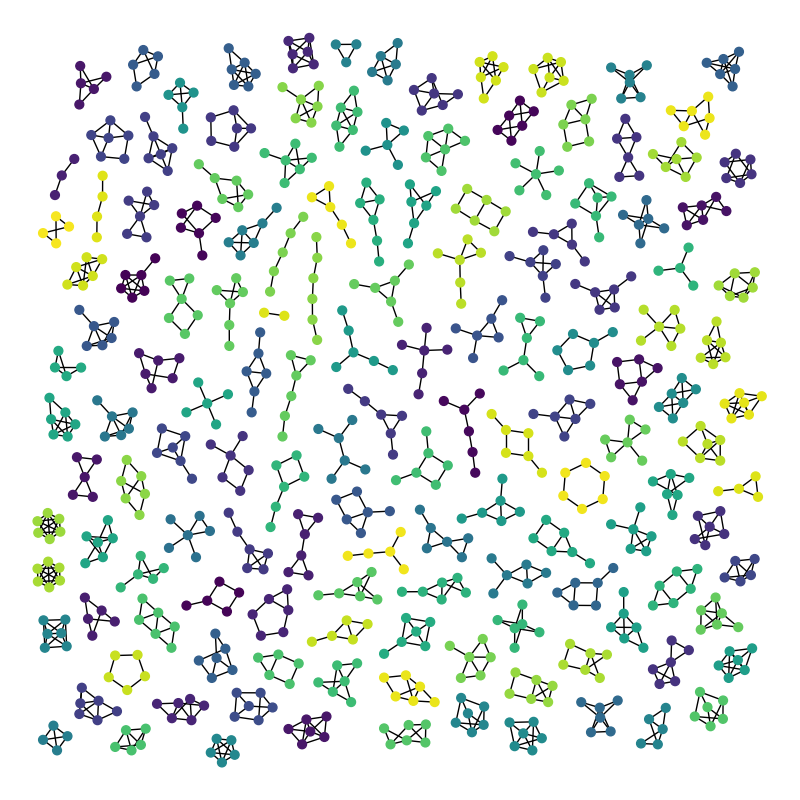# 阿特拉斯¶

6个节点及以下所有图形的图集。graph has 779 nodes with 1073 edges
137 connected components


# Author: Aric Hagberg (hagberg@lanl.gov)

#    Aric Hagberg <hagberg@lanl.gov>
#    Dan Schult <dschult@colgate.edu>
#    Pieter Swart <swart@lanl.gov>

import random

try:
import pygraphviz
from networkx.drawing.nx_agraph import graphviz_layout
except ImportError:
try:
import pydot
from networkx.drawing.nx_pydot import graphviz_layout
except ImportError:
raise ImportError("This example needs Graphviz and either "
"PyGraphviz or pydot.")

import matplotlib.pyplot as plt

import networkx as nx
from networkx.algorithms.isomorphism.isomorph import graph_could_be_isomorphic as isomorphic
from networkx.generators.atlas import graph_atlas_g

def atlas6():
""" Return the atlas of all connected graphs of 6 nodes or less.
Attempt to check for isomorphisms and remove.
"""

Atlas = graph_atlas_g()[0:208]  # 208
# remove isolated nodes, only connected graphs are left
U = nx.Graph()  # graph for union of all graphs in atlas
for G in Atlas:
zerodegree = [n for n in G if G.degree(n) == 0]
for n in zerodegree:
G.remove_node(n)
U = nx.disjoint_union(U, G)

# iterator of graphs of all connected components
C = (U.subgraph(c) for c in nx.connected_components(U))

UU = nx.Graph()
# do quick isomorphic-like check, not a true isomorphism checker
nlist = []  # list of nonisomorphic graphs
for G in C:
# check against all nonisomorphic graphs so far
if not iso(G, nlist):
nlist.append(G)
UU = nx.disjoint_union(UU, G)  # union the nonisomorphic graphs
return UU

def iso(G1, glist):
"""Quick and dirty nonisomorphism checker used to check isomorphisms."""
for G2 in glist:
if isomorphic(G1, G2):
return True
return False

if __name__ == '__main__':
G = atlas6()

print("graph has %d nodes with %d edges"
% (nx.number_of_nodes(G), nx.number_of_edges(G)))
print(nx.number_connected_components(G), "connected components")

plt.figure(1, figsize=(8, 8))
# layout graphs with positions using graphviz neato
pos = graphviz_layout(G, prog="neato")
# color nodes the same in each connected subgraph
C = (G.subgraph(c) for c in nx.connected_components(G))
for g in C:
c = [random.random()] * nx.number_of_nodes(g)  # random color...
nx.draw(g,
pos,
node_size=40,
node_color=c,
vmin=0.0,
vmax=1.0,
with_labels=False
)
plt.show()


Total running time of the script: ( 0 minutes 7.081 seconds)

Gallery generated by Sphinx-Gallery